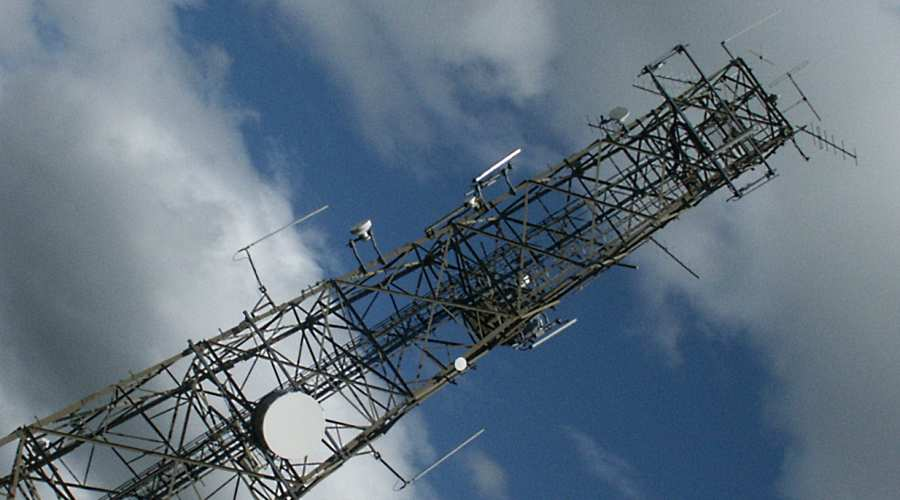The decibel is a way of expressing the relative value of something compared to a standard unit, often electrical power, or sound levels. In this case it is electrical power relative to a Watt. The decibel is a logarithmic unit, so 10dBW is ten Watts, 20dBW is a hundred Watts and 30dBW is a thousand Watts.

# Decibels relative to one Watt calculator

Enter either dB or Watt value then click Calculate button.

## Practical Application

Receiver sensitivity is usually expressed in dB's relative to a milliwatt or microwatt. The loss of energy as the radio signal propagates from transmit aerial to receive aerial is expressed in dB's. The use of dB's throughout the system makes it easy to calculate through a series of additions and subtractions if a certain signal could be received or not. To convert from dB relative to a Watt to dB relative to a milliwatt add 30. (e.g. 5dBW = 35dBm)

Calculator for dB milliwatts